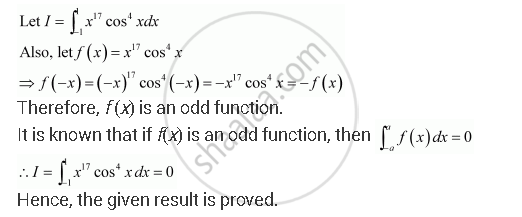Share

# Prove the Following Int_(-1)^1 X^17 Cos^4 Xdx = 0 - CBSE (Science) Class 12 - Mathematics

ConceptDefinite Integral as the Limit of a Sum

#### Question

Prove the following int_(-1)^1 x^17 cos^4 xdx = 0

#### SolutionIs there an error in this question or solution?

#### Video TutorialsVIEW ALL 

Solution Prove the Following Int_(-1)^1 X^17 Cos^4 Xdx = 0 Concept: Definite Integral as the Limit of a Sum.
S